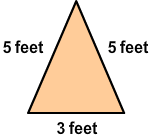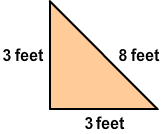# Area and Perimeter of Two Figures

Rate 0 stars Common Core
Quiz size:
Message preview:
Someone you know has shared Area and Perimeter of Two Figures quiz with you:

To play this quiz, click on the link below:

https://www.turtlediary.com/quiz/area-and-perimeter-of-two-figures.html

Hope you have a good experience with this site and recommend to your friends too.

Login to rate activities and track progress.
Login to rate activities and track progress.

The perimeter is the distance around the outside of an object. It is found by adding the lengths of all of the sides of the object together.

The area is the amount of surface enclosed by a closed figure.

Area of a rectangle = length × width

Area of a square = side × side

Using these formulae, we can also compare the area or perimeter of two given figures.

For example, consider the following figures:Let's compare their perimeters.

Perimeter of first figure = 5 + 3 + 5 = 13 feet

Perimeter of second figure = 3 + 8 + 3 = 14 feet

Now, 13 feet is less than 14 feet.

So, the perimeter of first figure is less than the perimeter of second figure.

ds

A B C D E F G H I J K L M N O P Q R S T U V W X Y Z